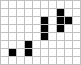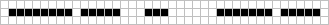# Infinite growth

A finite pattern is said to exhibit infinite growth if it is such that its population is unbounded. That is, for any number N there exists a generation n such that the population in generation n is greater than N. The first known pattern to exhibit infinite growth was the Gosper glider gun.

Apparent infinite growth may occur in many cellular automata, and rules in which such patterns are commonplace are known as class three cellular automata. However, in many cases there is no known proof that any such pattern in fact will continue to grow indefinitely (and is not simply a particularly vigorous methuselah). Most patterns known to be infinite growth patterns, therefore, follow some type of orderly periodic growth.

## Growth geometry

Infinite-growth patterns can be classified by their growth rate. In any cellular automaton, the maximum rate of growth is determined by the geometry of the space. In cellular automata set in 2D Euclidean space such as Life, the maximum growth rate is quadratic; cubic, quartic, quintic etc. growth require spaces of higher dimensionality.

For linear and quadratic growth patterns, S means stationary and M mobile.

### Linear

Four types of linear growth patterns can occur in cellular automata that operate by a basic engine that repeatedly creates new objects. In simple cases this leads to linear growth of the pattern's population:

• Type SS, a rare type of growth with both a stationary engine and output. One example of this is bricklayer.
• Type SM, a.k.a. gun: a pattern that has a stationary engine and moving output. For example, see Simkin glider gun.
• Type MS, a.k.a. puffer: a pattern that has a moving engine and stationary output. See puffer 2 for an example.
• Puffers whose output is a single, connected, growing object, and not isolated ash, are known as wickstretchers.
• Type MM, a.k.a. rake: a pattern that has a moving engine and moving output. Space rake is one such pattern.

A fifth type are linear replicators, which in their basic form cannot be separated into an engine and an output. Most known natural replicators have rule 90 type growth, and are thus sawtooths in their population growth rate.

Main article: Breeder

Quadratic growth can be split into these sections:

• SSS breeder - A stable object, usually a slide gun, creates copies of an SS infinite growth (e.g. a slide gun that shoots out a blockstacker-like mechanism).
• SSM breeder - A stable object that generates guns.
• SMS breeder - A stable object that generates puffers.
• SMM breeder - A stationary object that produces rakes. This is more commonly referred to as a rake gun.
• MSS breeder - A moving object that generates SS infinite growth.
• MMS breeder - A moving object generates puffers periodically. Examples include Riley's breeder, metacatacryst, Jaws and pufferfish breeder.
• MSM breeder - A puffer that produces guns. Gosper's original breeder was a MSM breeder; so was Lucas Brown's LWSS breeder.
• MMM breeder - A moving pattern that produces rakes periodically (i.e. a rake puffer).

The ones in bold require the use of slide guns, so are not very frequently constructed. The remaining four types are general types of breeders. However, breeders that can be classed as SSS and SMS have also been constructed.

Similarly to wickstretchers, the spacefiller is an infinite growth pattern that can be said to be MMS. This does not create puffers in the classical sense, but instead stretches an infinite line of wickstretchers.

## Small infinite growth patterns10-cell infinite growth
RLE: here

A natural question to ask is what the smallest starting size of an infinite growth pattern can be (either in terms of number of cells, bounding box or glider synthesis). In 1971, Charles Corderman found that a switch engine could be stabilized by a pre-block in a number of different ways to produce either a block-laying switch engine or a glider-producing switch engine, giving several 11-cell patterns with infinite growth. This record stood for more than quarter of a century until Paul Callahan found, in November 1997, two 10-cell patterns with infinite growth. The following month he found the one shown to the right, which is much neater, being a single cluster. It produces a block-laying switch engine. Today 24 different infinite growth patterns with 10 cells are known (most of them found by Michael Simkin in 2014). Nick Gotts and Paul Callahan have shown that there is no infinite growth pattern with fewer than 10 cells, so that the question of the smallest infinite growth pattern in terms of number of cells has been answered completely.

On October 24, 2014, Michael Simkin disovered a three-glider collision for a pattern whose ash includes a glider-producing switch engine.

Also of interest are some infinite growth patterns with particularly small bounding boxes. The following pattern is the smallest one-cell-thick pattern that exhibits infinite growth, found via computer search in October 1998 by Callahan:Paul Callahan's one cell thick infinite growth pattern

Indeed, this pattern produces two block-laying switch engines at about generation 700. The following pattern (also found by Callahan) is the only pattern with infinite growth that fits inside a 5×5 bounding box. It too emits a block-laying switch engine.Paul Callahan's 5×5 infinite growth pattern
RLE: here

Paul Callahan's pattern shows that infinite growth patterns exist in bounding boxes with area 25, but whether or not infinite growth patterns could exist in smaller boxes was not known until 2009, when exhaustive computer searches were conducted to show that there is an infinite growth pattern with bounding box 2×12 (area 24), and that this area is minimal. This pattern is shown below.The minimally sized 2×12 infinite growth pattern
RLE: here

## Growth rates

Although the simplest infinite growth patterns grow at a rate that is (asymptotically) linear, many other growth rates are possible. The following table summarizes asymptotic growth rates that have been explicitly constructed in Life:

Growth rate f(t) Examples
t2 (quadratic) breeder 1, max, mosquito 5, metacatacryst
t3/2 ?
tlog(t)2 ?
tlog(t) tlog(t) growth, Gotts dots
tlog(log(t)) tlog(log(t)) growth
tlog*(n)(t) Sawmill
t (linear) block-laying switch engine, Gosper glider gun, space rake, puffer 2
sqrt(t) sqrtgun
t1/3 ?
t^(2^(-n)) ?
log(t)2 log(t)^2 growth
log(t) (logarithmic) Caber tosser 1
log(n)(t) ?
log*(n)(t) Sawmill

It is not difficult to see that quadratic growth is the fastest possible growth rate, and many patterns that grow at such speed are now known. There are patterns that exhibit infinite growth but whose population does not tend toward infinity – see sawtooth. By combining a sawtooth with a pattern that grows infinitely at a different rate, it is possible to construct patterns that grow (for example) logarithmically at some times and linearly at other times. There are even patterns, such as the Fermat prime calculator, for which it is not known if they grow infinitely or not.